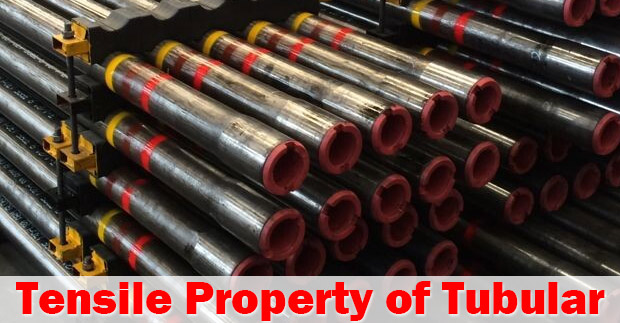# Tesile Property of Pipe

Oil field tubular is typically designed to work under its minimum yield strength so it means that the tubular will work with tensile load within the elastic limit. This article will discuss about tensile properties and how to determine tensile of oilfield tubular.Tensile strength of tubular can be calculated by this following equation.

## Tensile strength = Minimum Yield Strength × Cross Sectional Area

Where;

Tensile strength in lb

Minimum yield strength in psi

Cross sectional area in in2Figure 1 – Tensile Diagram

Minimum yield strength of pipe can be found in the grade of the string. For example, the pipe grade if J-55 has minimum yield strength of 55,000 psi. The number after alphabet represents the minimum yield strength in 1,000 psi. A few examples are shown in the Figure 2.Figure 2 – Numbers represents pipe minimum yield strength

API5CT specifies pipe specification as shown in the Figure 3.Figure 3 – API Steel Grade Table

Example: Calculate the tensile strength of the following pipe.

4-1/2” casing, weight 9.5 ppf,  grade J-55

Pipe ID = 4.09”

Cross sectional area (in2) = (π÷4) × (OD2 – ID2)

Cross sectional area (in2) = (π÷4) × (4.52 – 4.092)

Cross sectional area (in2) = 2.77 in2

Minimum Tensile Strength (lb) = 55,000 × 2.77 = 152 Klb

This number can be found in the API pipe specification.Figure 4 – API Tubular Specification

References

Jonathan Bellarby, 2009. Well Completion Design, Volume 56 (Developments in Petroleum Science). 1 Edition. Elsevier Science.

Wan Renpu, 2011. Advanced Well Completion Engineering, Third Edition. 3 Edition. Gulf Professional Publishing.

Ted G. Byrom, 2014. Casing and Liners for Drilling and Completion, Second Edition: Design and Application (Gulf Drilling Guides). 2 Edition. Gulf Professional Publishing.

Share the joy
Tagged , , , . Bookmark the permalink.